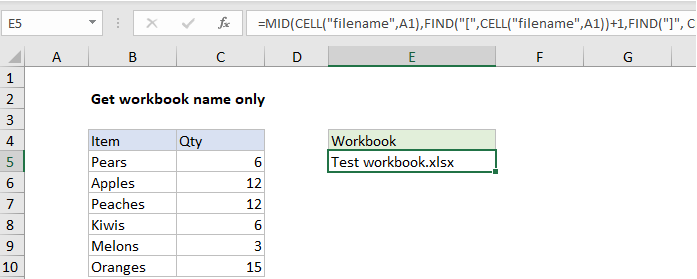## Excel Office

Excel How Tos, Tutorials, Tips & Tricks, Shortcuts

# How to retrieve workbook name only in Excel

If you want to get the workbook name only (i.e. the file name without path or sheet name) you can do so with a rather long formula that uses the MID function along with the FIND function.

Worked Example:   Extract middle name from full name -- Manipulating NAMES in Excel

## Formula

```=MID(CELL("filename",A1),FIND("[",CELL
("filename",A1))+1,FIND("]",CELL("filename",A1))
-FIND("[",CELL("filename",A1))-1)```## Explanation

How the formula works

The cell function is used to get the full file name and path:

`CELL("filename",A1)`

The result looks like this:

`path[workbook.xlsm]sheetname`

At the highest level, the MID function simply extracts the file name from the path and sheet. The starting position is calculated with FIND:

`FIND("[",CELL("filename",A1))+1`

The number of characters to extract is also calculated with FIND:

`FIND("]",CELL("filename",A1))-FIND("[",CELL("filename",A1))-1`